# Functions + analytic geometry - math problems

#### Number of problems found: 21

• Ascend vs. descentWhich function is growing? a) y = 2-x b) y = 20 c) y = (x + 2). (-5) d) y = x-24 m long ladder touches the cube 1mx1m at the wall. How high reach on the wall?
• Find the 15Find the tangent line of the ellipse 9 x2 + 16 y2 = 144 that has the slope k = -1
• Linear independence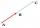Determine if vectors u=(-4; -5) and v=(20; 25) are linear Linear dependent.
• Symmetry by plane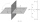Determine the coordinates of a image of point A (3, -4, -6) at a symmetry that is determined by the plane x-y-4z-13 = 0
• Slope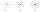Calculate the slope of a line that intersects points (-84,41) and (-76,-32).
• V - slopeThe slope of the line whose equation is -3x -9 = 0 is
• Tangents to ellipseFind the magnitude of the angle at which the ellipse x2 + 5 y2 = 5 is visible from the point P[5, 1] .
• Lie/do not lie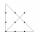The function is given by the rule f(x) = 8x+16. Determine whether point D[-1; 8] lies on this function. Solve graphically or numerically and give reasons for the your answer.
• CircleCircle is given by centre on S[-7; 10] and maximum chord 13 long. How many intersect points have circle with the coordinate axes?
• A screenA screen is 1680 x 1050 pixels. What are the coordinates (and size in pixels) of an centered area which is exactly 33% of the screen size?
• Line segmentFor the line segment whose endpoints are L[-1, 13] and M[18, 2], find the x and y value for the point located 4 over 7 the distance from L to M.
• Set of coordinatesConsider the following ordered pairs that represent a relation. {(–4, –7), (0, 6), (5, –3), (5, 2)} What can be concluded of the domain and range for this relation?
• Three vectorsThe three forces whose amplitudes are in ratio 9:10:17 act in the plane at one point to balance. Determine the angles of each two forces.
• Points on line segmentPoints P & Q belong to segment AB. If AB=a, AP = 2PQ = 2QB, find the distance: between point A and the midpoint of the segment QB.
• Coordinates of square verticesI have coordinates of square vertices A / -3; 1/and B/1; 4 /. Find coordinates of vertices C and D, C 'and D'. Thanks Peter.
• General line equationsIn all examples, write the GENERAL EQUATION OF a line that is given in some way. A) the line is given parametrically: x = - 4 + 2p, y = 2 - 3p B) the line is given by the slope form: y = 3x - 1 C) the line is given by two points: A [3; -3], B [-5; 2] D) t
• Points in space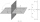There are n points, of which no three lie on one line and no four lies on one plane. How many planes can be guided by these points? How many planes are there if there are five times more than the given points?
• Curve and lineThe equation of a curve C is y=2x² -8x+9 and the equation of a line L is x+ y=3 (1) Find the x co-ordinates of the points of intersection of L and C. (2) Show that one of these points is also the stationary point of C?
• ProveProve that k1 and k2 are the equations of two circles. Find the equation of the line that passes through the centers of these circles. k1: x2+y2+2x+4y+1=0 k2: x2+y2-8x+6y+9=0

Do you have an interesting mathematical word problem that you can't solve it? Submit a math problem, and we can try to solve it.

We will send a solution to your e-mail address. Solved examples are also published here. Please enter the e-mail correctly and check whether you don't have a full mailbox.

Please do not submit problems from current active competitions such as Mathematical Olympiad, correspondence seminars etc...

For Basic calculations in analytic geometry is a helpful line slope calculator. From coordinates of two points in the plane it calculate slope, normal and parametric line equation(s), slope, directional angle, direction vector, the length of segment, intersections the coordinate axes etc. Functions - math word problems. Analytic geometry - math word problems.